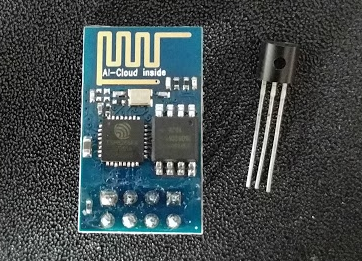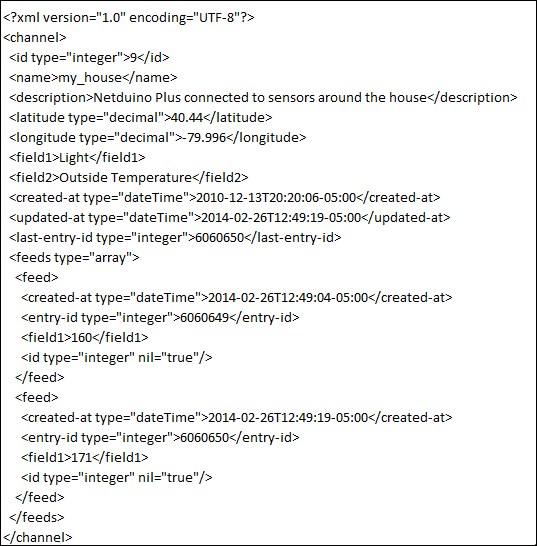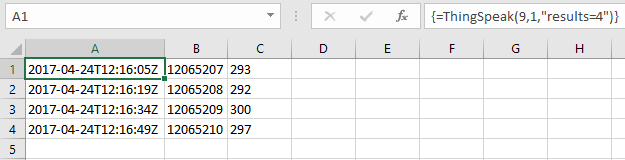Author: Oscar Cronquist Article last updated on July 25, 2017

Thingspeak is web service that allows you to upload and store data from IoT devices.Picture above shows cheap chinese IoT modules: a temp sensor and an esp8266-01 (25 mm x 15 mm)

Today I want to demonstrate a user defined function that downloads data from ThingSpeak to Excel so you can easily analyze and chart the data yourself, in excel.

The way this works is that using a simple http address with your channel and field number and parameters, you can easily fetch values from your feed.

The http address returns xml data, something like this:The following user defined function lets you specify a channel, field and some more parameters. The function then removes xml tags and returns data to worksheet.Remember to enter this as an array formula, 3 columns wide.

```Function ThingSpeak(ch As Long, fld As Integer, prm As String)
If prm <> "" Then prm = "?" & prm
'Build url
url = "https://api.thingspeak.com/channels/" & ch & "/fields/" & fld & ".xml" & prm
On Error Resume Next
Set http = CreateObject("MSXML2.XMLHTTP")
http.Open "GET", url, False
http.Send
tmp = http.responseText
'Check for errors
If Err <> 0 Then
MsgBox "Error fetching values"
On Error GoTo 0
Exit Function
End If
On Error GoTo 0

'Remove beginning xml data
tmp = Right(tmp, Len(tmp) - InStr(tmp, "") + 1)

'Clear xml tags with RemoveFromStr udf and then return values to worksheet
ThingSpeak = RemoveFromStr(tmp)

End Function
```
```Function RemoveFromStr(tmp As Variant)
Dim tmp2 As Variant
'Clear xml tags using a regular expression
regexpattern = "<.*?>"
With CreateObject("vbscript.regexp")
.Global = True
.MultiLine = True
.IgnoreCase = False
.Pattern = regexpattern
Set Results = .Execute(tmp)
End With
If Results.Count <> 0 Then
With Results
For d = 0 To .Count - 1
tmp = Replace(tmp, .Item(d), ",")
Next
End With
End If

'Remove spaces
tmp = Replace(tmp, " ", "")

'Remove newline
tmp = Replace(tmp, vbLf, "")

'Replace T and Z with space character
tmp = Replace(tmp, "T", " ")
tmp = Replace(tmp, "Z", " ")

'Combine multiple commas
For i = 1 To Len(tmp)
If Mid(tmp, i, 1) = "," And Mid(tmp, i, 1) = Mid(tmp, i + 1, 1) Then
Else
tmp1 = tmp1 & Mid(tmp, i, 1)
End If
Next i

'Remove beginning and ending commas
If Left(tmp1, 1) = "," Then tmp1 = Right(tmp1, Len(tmp1) - 1)
If Right(tmp1, 1) = "," Then tmp1 = Left(tmp1, Len(tmp1) - 1)

'Split text into 1D array
tmp1 = Split(tmp1, ",")

'Count array
j = ((UBound(tmp1) - LBound(tmp1) + 1) / 3) - 1

'Build 2D array
ReDim tmp2(0 To j, 0 To 2)
ro = 0
co = 0
For i = LBound(tmp1) To UBound(tmp1)
tmp2(ro, co) = tmp1(i)
co = co + 1
If co = 3 Then
co = 0
ro = ro + 1
End If
Next i
'Return array
RemoveFromStr = tmp2

End Function

```